# LAB 8.2 WHILE Loops

 Team-Fly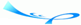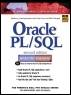Oracle® PL/SQL® Interactive Workbook, Second EditionBy Benjamin Rosenzweig, Elena Silvestrova Table of Contents Chapter 8.  Iterative Control

Lab Objectives

After this Lab, you will be able to:

• Use WHILE Loops

A WHILE loop has the following structure:

` WHILE CONDITION LOOP     STATEMENT 1;     STATEMENT 2;     …     STATEMENT N;  END LOOP; `

The reserved word WHILE marks the beginning of a loop construct. The word CONDITION is the test condition of the loop that evaluates to TRUE or FALSE. The result of this evaluation determines whether the loop is executed. Statements 1 through N are a sequence of statements that is executed repeatedly. The END LOOP is a reserved phrase that indicates the end of the loop construct.

This flow of the logic is illustrated in Figure 8.3.

##### Figure 8.3. WHILE Loop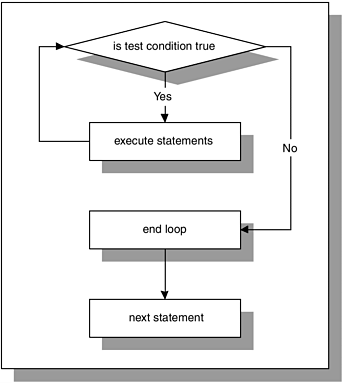Figure 8.3 shows that the test condition is evaluated prior to each iteration of the loop. If the test condition evaluates to TRUE, the sequence of statements is executed, and control is passed to the top of the loop for the next evaluation of the test condition. If the test condition evaluates to FALSE, the loop is terminated, and control is passed to the next executable statement following the loop.

As mentioned earlier, before the body of the loop can be executed, the test condition must be evaluated. The decision as to whether to execute the statements in the body of the loop is made prior to entering the loop. As a result, the loop will not be executed at all if the test condition yields FALSE.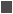FOR EXAMPLE

` DECLARE     v_counter NUMBER := 5;  BEGIN     WHILE v_counter < 5 LOOP        DBMS_OUTPUT.PUT_LINE ('v_counter = '||v_counter);        -- decrement the value of v_counter by one        v_counter := v_counter - 1;     END LOOP;  END; `

In this example, the body of the loop is not executed at all because the test condition of the loop evaluates to FALSE.

While the test condition of the loop must evaluate to TRUE at least once for the statements in the loop to execute, it is important to insure that the test condition will eventually evaluate to FALSE, as well. Otherwise, the WHILE loop will execute continually.FOR EXAMPLE

` DECLARE     v_counter NUMBER := 1;  BEGIN     WHILE v_counter < 5 LOOP        DBMS_OUTPUT.PUT_LINE('v_counter = '||v_counter);        -- decrement the value of v_counter by one        v_counter := v_counter - 1;     END LOOP;  END; `

This is an example of an infinite WHILE loop. The test condition always evaluates to TRUE, because the value of v_counter is decremented by 1 and is always less than 5.It is important to note that Boolean expressions can also be used to determine when the loop should terminate.

` DECLARE     v_test BOOLEAN := TRUE;  BEGIN     WHILE v_test LOOP        STATEMENTS;        IF TEST_CONDITION THEN           v_test := FALSE;        END IF;     END LOOP;  END; `

When using a Boolean expression as a test condition of a loop, you must make sure that a different value is eventually assigned to the Boolean variable in order to exit the loop. Otherwise, the loop will become infinite.

#### Premature Termination of the Loop

The EXIT and EXIT WHEN statements can be used inside the body of a WHILE loop. If the EXIT condition evaluates to TRUE before the test condition evaluates to FALSE, the loop is terminated prematurely. If the test condition yields FALSE before the EXIT condition yields TRUE, there is no premature termination of the loop. This is indicated as follows:

` WHILE TEST_CONDITION LOOP     STATEMENT 1;     STATEMENT 2;     IF EXIT_CONDITION THEN        EXIT;     END IF;  END LOOP;  STATEMENT 3; `

or

` WHILE TEST_CONDITION LOOP     STATEMENT 1;     STATEMENT 2;     EXIT WHEN EXIT_CONDITION;  END LOOP;  STATEMENT 3; `

Consider the following example.FOR EXAMPLE

` DECLARE     v_counter NUMBER := 1;  BEGIN     WHILE v_counter <= 5 LOOP         DBMS_OUTPUT.PUT_LINE ('v_counter = '||v_counter);        IF v_counter = 2 THEN           EXIT;        END IF;        v_counter := v_counter + 1;     END LOOP;  END; `

Before the statements in the body of the WHILE loop are executed, the test condition

` v_counter <= 5 `

must evaluate to TRUE. Then, the value of v_counter is displayed on the screen and incremented by one. Next, the EXIT condition

` v_counter = 2 `

is evaluated, and as soon as the value of v_counter reaches 2, the loop is terminated.

Notice that according to the test condition, the loop should execute five times. However, the loop is executed only twice, because the EXIT condition is present inside the body of the loop. Therefore, the loop terminates prematurely.

Now you will try to reverse the test condition and EXIT condition.FOR EXAMPLE

` DECLARE     v_counter NUMBER := 1;  BEGIN     WHILE v_counter <= 2 LOOP        DBMS_OUTPUT.PUT_LINE ('v_counter = '||v_counter);        v_counter := v_counter + 1;        IF v_counter = 5 THEN           EXIT;        END IF;     END LOOP;  END; `

In this example, the test condition is

` v_counter <= 2 `

and the EXIT condition is

` v_counter = 5 `

In this case, the loop is executed twice as well. However, it does not terminate prematurely, because the EXIT condition never evaluates to TRUE. As soon as the value of v_counter reaches 3, the test condition evaluates to FALSE, and the loop is terminated.

Both examples, when run, produce the following output:

` v_counter = 1  v_counter = 2  PL/SQL procedure successfully completed. `

These examples demonstrate not only the use of the EXIT statement inside the body of the WHILE loop, but also a bad programming practice. In the first example, the test condition can be changed so that there is no need to use an EXIT condition, because essentially they both are used to terminate the loop. In the second example, the EXIT condition is useless, because its terminal value is never reached. You should never use unnecessary code in your program.

#### Lab 8.2 Exercises

##### 8.2.1 Use WHILE Loops

In this exercise, you will use a WHILE loop to calculate the sum of the integers between 1 and 10.

Create the following PL/SQL script:

` -- ch08_3a.sql, version 1.0  SET SERVEROUTPUT ON  DECLARE     v_counter BINARY_INTEGER := 1;     v_sum NUMBER := 0;  BEGIN     WHILE v_counter <= 10 LOOP        v_sum := v_sum + v_counter;        DBMS_OUTPUT.PUT_LINE ('Current sum is: '||v_sum);        -- increment loop counter by one        v_counter := v_counter + 1;     END LOOP;     -- control resumes here     DBMS_OUTPUT.PUT_LINE ('The sum of integers between 1 '||        'and 10 is: '||v_sum);  END; `

Execute the script, and then answer the following questions:

 a) What output was printed on the screen? b) What is the test condition for this loop? c) How many times was the loop executed? d) How many times will the loop be executed if v_counter is not initialized?if v_counter is initialized to 0?if v_counter is initialized to 10? e) How will the value of v_sum change based on the initial value of v_counter from the previous question? f) What will be the value of v_sum if it is not initialized? g) How would you change the script to calculate the sum of the even integers between 1 and 100?

#### Lab 8.2 Exercise Answers

This section gives you some suggested answers to the questions in Lab 8.2, with discussion related to how those answers resulted. The most important thing to realize is whether your answer works. You should figure out the implications of the answers here and what the effects are from any different answers you may come up with.

##### 8.2.1 Answers

 a) What output was printed on the screen? A1: Answer: Your output should look like the following:` Current sum is: 1 Current sum is: 3 Current sum is: 6 Current sum is: 10 Current sum is: 15 Current sum is: 21 Current sum is: 28 Current sum is: 36 Current sum is: 45 Current sum is: 55 The sum of integers between 1 and 10 is: 55 PL/SQL procedure successfully completed. `Every time the loop is run, the value of v_counter is checked in the test condition. While the value of v_counter is less than or equal to 10, the statements inside the body of the loop are executed. In this script, the value of v_sum is calculated and displayed on the screen. Next, the value of v_counter is incremented, and control is passed to the top of the loop. Once the value of v_counter increases to 11, the loop is terminated.For the first iteration of the loop, the value of v_sum is equal to 1, according to the statement` v_sum := v_sum + v_counter `After the value of v_sum is calculated, the value of v_counter is incremented by 1. Then, for the second iteration of the loop, the value of v_sum is equal to 3, because 2 is added to the old value of v_sum.After the loop has terminated, "The sum of integers..." and "Done ..." are displayed on the screen. b) What is the test condition for this loop? A2: Answer: The test condition for this loop is v_counter <= 10. c) How many times was the loop executed? A3: Answer: The loop was executed 10 times.Once the value of v_counter reaches 11, the test condition` v_counter <= 10 `evaluates to FALSE, and the loop is terminated.As mentioned earlier, the loop counter tracks the number of times the loop is executed. You will notice that in this exercise, the maximum value of v_counter is equal to the number of times the loop is iterated. d) How many times will the loop be executedif v_counter is not initialized?if v_counter is initialized to 0?if v_counter is initialized to 10? A4: Answer: If the value of v_counter is not initialized to some value, the loop will not execute at all.In order for the loop to execute at least once, the test condition must evaluate to TRUE at least once. If the value of v_counter is only declared and not initialized, it is NULL. It is important to remember that null variables cannot be compared to other variables or values. Therefore, the test condition` v_counter <= 10 `never evaluates to TRUE, and the loop is not executed at all.If v_counter is initialized to 0, the loop will execute 11 times instead of 10, since the minimum value of v_counter has decreased by 1.When v_counter is initialized to 0, the range of integers for which the test condition of the loop evaluates to TRUE becomes 0 to 10. The given range of the integers has eleven numbers in it. As a result, the loop will iterate eleven times.If v_counter is initialized to 10, the loop will execute once.When the initial value of v_counter is equal to 10, the test condition evaluates to TRUE for the first iteration of the loop. Inside the body of the loop, the value of v_counter is incremented by one. As a result, for the second iteration of the loop, the test condition evaluates to FALSE, since 11 is not less than or equal to 10, and control is passed to the next executable statement after the loop. e) How will the value of v_sum change based on the initial value of v_counter from the previous question? A5: Answer: When v_counter is not initialized, the loop is not executed at all. Therefore, the value of v_sum does not change from its initial value; it stays 0.When v_counter is initialized to 0, the loop is executed 11 times. The value of v_sum is calculated 11 times, as well. However, after the loop completes, the value of v_sum is 55, because 0 is added to v_sum during first iteration of the loop.When v_counter is initialized to 10, the loop is executed once. As a result, the value of v_sum is incremented only once by 10. After the loop is complete, the value of v_sum is equal to 10. f) What will be the value of v_sum if it is not initialized? A6: Answer: The value of v_sum will be NULL if is not initialized to some value.The value of v_sum in the statement` v_sum := v_sum + 1 `will always be equal to NULL, because NULL + 1 is NULL. It was mentioned earlier that NULL variables cannot be compared to other variable or values. Similarly, calculations cannot be performed on null variables. g) How would you change the script to calculate the sum of the even integers between 1 and 100? A7: Answer: Your answer should be similar to the following. Changes are shown in bold letters.Notice that the value of v_counter is initialized to 2, and with each iteration of the loop, the value of v_counter is incremented by 2, as well.` -- ch08_3b.sql, version 2.0 SET SERVEROUTPUT ON DECLARE v_counter BINARY_INTEGER := 2; v_sum NUMBER := 0; BEGIN WHILE v_counter <= 100 LOOP v_sum := v_sum + v_counter; DBMS_OUTPUT.PUT_LINE ('Current sum is: '||v_sum); -- increment loop counter by two v_counter := v_counter + 2; END LOOP; -- control resumes here DBMS_OUTPUT.PUT_LINE ('The sum of even integers between '||'1 and 100 is: '||v_sum); END; `

#### Lab 8.2 Self-Review Questions

In order to test your progress, you should be able to answer the following questions.

Answers appear in Appendix A, Section 8.2.

 1) How many times is a WHILE loop executed if the test condition always evaluates to FALSE? _____ The loop does not execute at all._____ The loop executes once._____ The loop executes an infinite number of times. 2) How many times is a WHILE loop executed if the test condition always evaluates to TRUE? _____ The loop does not execute at all._____ The loop executes once._____ The loop executes an infinite number of times. 3) What value must the test condition evaluate to in order for the loop to terminate? _____ TRUE_____ FALSE_____ NULL 4) What causes a WHILE loop to terminate prematurely? _____ The EXIT condition evaluates to TRUE before the test condition evaluates to FALSE._____ The test condition evaluates to FALSE before the EXIT condition evaluates to TRUE._____ Both test and EXIT conditions evaluate to FALSE. 5) A WHILE loop will execute a minimum of _____ zero times._____ one time._____ infinite number of times.

 Team-FlyTop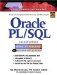Oracle PL/SQL Interactive Workbook (2nd Edition)
ISBN: 0130473200
EAN: 2147483647
Year: 2002
Pages: 146

Similar book on Amazon

flylib.com © 2008-2017.
If you may any questions please contact us: flylib@qtcs.net